# RD Sharma Solutions Chapter 2 Polynomials Exercise 2.1 Class 10 Maths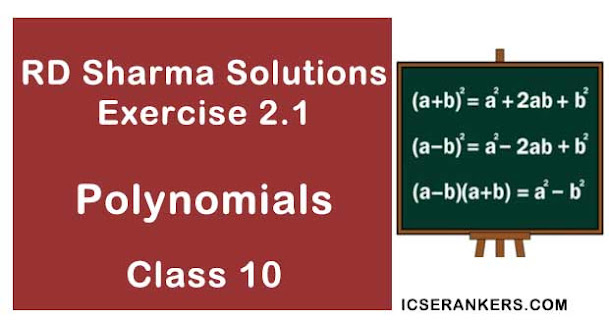Chapter Name RD Sharma Chapter 2 Polynomials Book Name RD Sharma Mathematics for Class 10 Other Exercises Exercise 2.2Exercise 2.3 Related Study NCERT Solutions for Class 10 Maths

### Exercise 2.1 Solutions

1. Find the zeroes of each of the following quadratic polynomials and verify the relationship between the zeroes and their co efficient :
(i) f(x) = x2 – 2x – 8
(ii) g(s) = 4s2 – 4x + 1
(iii) h(t) = t2 – 15
(iv) p(x)  = x2 + 2√2x + 6
(v) q(x) = √3x2 + 10x + 7√3
(vi) f(x) = x2 – (√3 + 1)x + √3
(vii) g(x) = a(x2 + 1) – x(a2 + 1)
(viii) 6x2 – 3 – 7x

Solution

(i) f(x) = x2 – 2x – 8
f(x) = x2 – 2x – 8 = x2 – 4x + 2x – 8
= x(x – 4) + 2(x – 4)
= (x + 2) (x – 4)
Zeroes of the polynomials are –2 and 4
Sum of the zeroes = –(co efficient of x)/(co efficient of x)
– 2+ 4 = – (–2)/1
⇒ 2 = 2
Product of the zeroes = (constant term)/(co efficient of x2 )
= 24 = –8/1
⇒ –8 = –8
∴ Hence the relationship verified

(ii) 9(5) = 45 - 45 + 1 = 452 - 25 - 25 + 1 = 25(25 - 1) - 1(25 - 1)
= (25 - 1)(25 - 1 )
Zeroes of the polynomials are 1/2 and 1/2
Sum of zeroes = (–co efficient of s)/(co efficient of s2 )
1/2 + 1/2 = -(-4)/4
⇒ 1 = 1
Product of the zeroes = (constant term)/(co efficient of s2 )
1/2 ×1/2 = 1/4

⇒ 1/4 = 1/4
∴ Hence the relationship verified.

(iii) h(t)  = t2 - 15 = (t2) - (√15)2 = (t + √15)(t - √15)
zeroes of the polynomials are  -√15 and √15
sum of zeroes  = 0
- √15 + √15 = 0
⇒ 0 = 0
Product of zeroes = -15/1
-√15 × √15 = - 15
⇒ -15 = -15
∴ Hence the relationship verified.

(iv) p(x) = x2  + 2√2x - 6  = x2 + 3√2x + √2 × 3√2
= x(x + 3√2) - √2( 2 + 3√2) = (x - √2)(x + 3√2)
Zeroes of the polynomial are 3√2 and -3√2
Sum of the zeroes = -3√2/1
√2 - 3√2 = -2√2
⇒ -2√2 = -2√2
Product of zeroes = √2

⇒ √2 × -3√2 = -6/1
-6 = -6
Hence, the relationship verified.

(v) 2(x)  = √3x2  + 10x + 7√3 = √3x2  + 7x + 3x + 7√3
= √3x(x + √3) + 7(x +√3)
= (√3x + 7)(x + √3)
Zeroes of the polynomials are - √3, -7/√3
Sum of zeroes = -10/√3
⇒ -√3 - 7/√3  = -10/√3

Product of zeroes  = 7√3/3

⇒ (√3x - 7)/√30 = 7
⇒ 7 = 7
Hence, relationship verified.

(vi) f(x) = x2 - (√3 + 1)x + √3 = x2  - √3x - x +  √3
= x(x - √3) - 1(x - √3)
= (x - 1)(x - √3)
Zeroes of the polynomials are 1 and √3
Sum of zeroes = -( co efficient of x)/(co efficient x2) = -(-√3-1)/1
1 + √3 = √3 +1
Product of zeroes = (constant term)/(co efficient of x2) = √3/1
1 × √3  = √3  = √3  = √3
∴ Hence, relationship verified.

(vii) g(x) = a[(x2 + 1) - x(a2 + 1)]2 = ax2 + a - a2 x - x
= ax2 - [(a2 + 1) -x]  + 0 = ax2  - a2 x - x + a
= ax(x -a) - 1(x-a) = (x - a)(ax - 1)
Zeroes of the polynomials  = 1/a and a
Sum of the zeroes  = -[-a2 - 1]/a
⇒ 1/a + a = (a2  + 1)/a

⇒ (a2 + 1)/a = (a2 + 1)/a
Product of zeroes = a/a
⇒ 1/a × a = a/a

⇒ (a2 + 1)/a = (a2 + 1)/a
Product of zeroes = a/a

⇒ 1 = 1
Hence, relationship verified

(viii) 6x2 - 3 - 7x

= 6x2 - 7x - 3

= (3x + 11)(2x - 3)
Zeroes of polynomials are  +3/2 and -1/3
Sum of zeroes = -1/3 + 3/2

= 7/6

= -(-7)/6

= -(co efficeint of x)/(co efficient of x2 )
Product of zeroes = -1/3 × 3/2

= -1/2

= -3/6

= (constant term)/(co efficient of x2 )
∴ Hence, relationship verified.

2. If α and β are the zero of the quadratic polynomial f(x) = ax2 + bx + c, then evaluate:
(i) α - β
(ii) 1/α - 1/ß
(iii) 1/α + 1/ß - 2αß
(iv) α2 ß+ αß2
(v) α4 + ß4
(vi) 1/(aα + b) + 1/(aß + b)
(vii) ß/(aα+b) + α/(aß+b)
(viii) a[α2/ß + ß2/α] + b[α/a + ß/a]

Solution

f(x) = ax2  + bx + c
α + ß = -b/a
αß = c/a
since α + ß are the roots (or) zeroes of the given polynomials

(i) α - ß
The two zeroes of the polynomials are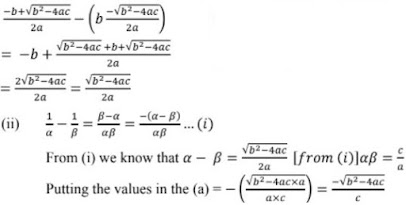(iii) 1/α + 1/ß - 2αß
⇒ [(α+ß)/αß] - 2αß
⇒ -b/a × a/c - 2c/a

= -2c/a - b/c

= (-ab - 2c2)/ac - [b/c + 2c/a]

(iv) a2ß + αß2
αß(α + ß)
= c/a(-b/a)
= -bc/a2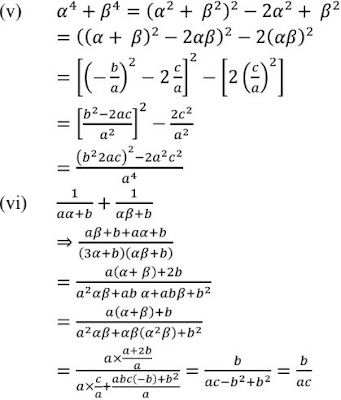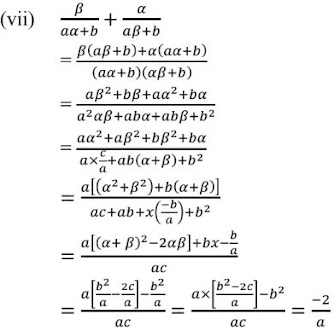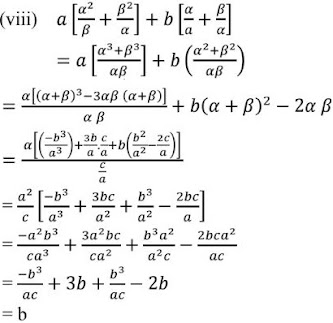3. If α and ß are the zeros of the quadratic polynomial f(x) = 6x2 + x - 2, find the value of α/ß + ß/α
Solution
f(x)  = 6x2 - x - 2
Since α and ß are the zeroes of the given polynomial
∴ Sum of zeroes [α + ß] = -1/6
Product of zeroes  (αß) =  - 1/3
= α/ß + ß/α = (α2 + ß2 )/αß = [(α+ß)2 - 2αß]/αß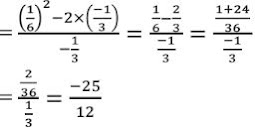4. If α and ß are the zeros of the quadratic polynomial f(x) = x2 - x + 4, find the value of 1/α + 1/ß - αß.
Solution

Since α + ß are the zeroes of the polynomial : x2 - x - 4
Sum of the roots (α + ß) = 1
Product of the roots(αß) = -4
1/α + 1/ß - αß = (α+ß)/αß - αß
= 1/-4 + 4

= -1/4 + 4

= (-1 + 16)/4

= 15/4

5. If α and ß  are the zeros of the quadratic polynomial p(x) = 4x2 - 5x - 1, find the value of α2 ß + αß2.
Solution

Since α and ß are the roots of the polynomial : 4x2 - 5x - 1
∴ Sum of the roots α + ß = 5/4
Product of the roots αß = -1/4
Hence α2ß + αß2 = αß(α + ß) = 5/4(-1/4) = -5/16

6. If α and ß are the zeros of the quadratic polynomial f(x) = x2 + x - 2, find the value of 1/α - 1/ß.
Solution

Since α and ß are the roots of the polynomial x2  + x - 2

∴ Sum of roots α + ß = 1
Product of roots αß = -2
⇒ -1/ß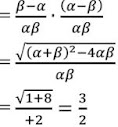7. If α and ß are the zeros of the quadratic polynomial f(x) = x2 - 5x + 4, find the value of 1/α - 1/ß - 2αß.
Solution

Since α and ß are the roots of the quadratic polynomial
f(x)= x2 - 5x + 4
Sum of roots  = α + ß = 5
Product of roots = αß = 4
1/α  + 1/ß - 2αß

= (ß+α)/αß - 2αß

= 5/4 - 2× 4

= 5/4 - 8

= -27/4

8. If α and ß  are the zeros of the quadratic polynomial f(t) = t2  - 4t + 3, find the value of α4ß3 + α3ß4 .
Solution

Since α and ß are the zeroes of the polynomial f(t) = t2  - 4t + 3
Since α + ß = 4
Product of zeroes αß = 3
Hence α4ß3  + α3ß4 = α3ß3 (α + ß) = 2  = 108

9. If α and ß are the zeros of the quadratic polynomial p(y) = 5y2 - 7y + 1, find the value of 1/α + 1/ß.
Solution

Since α and ß are the zeroes of the polynomials
p(y) = 5y2 - 7y + 1
Sum of the zeroes αß = 1/6
Product of zeroes αß = 1/6
1/α + 1/ß

= (α+ß)/αß

= (7×5)/(5×1)

= 7

10. If α and ß are the zeros of the quadratic polynomial p(s) = 3s2 - 6s + 4, find the value of α+ß + ß/α + 2[1/α + 1/ß] + 3αß
Solution

Since α and ß are the zeroes of the polynomials
Sum of the zeroes α + ß = 6/3
Product of the zeroes αß = 4/3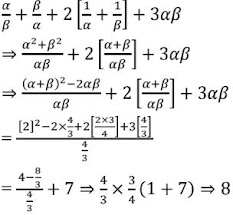11. If α and ß are the zeros of the quadratic polynomial f(x) = x2 - px + q, prove that
α22 + ß2 + α2 = p2/q2 - 4p2/q + 2
Solution

Since α and ß are the roots of the polynomials
f(x)  = x2 - px + 2
sum of zeroes = p = α + ß
Product of zeroes = q = αß12. If the squared difference of the zeros of the quadratic polynomial f(x) = x2 + px + 45 is equal to 144. Find the value of p.
Solution

Let the two zeroes of the polynomial be α and ß
f(x) = x2 + px + 45
sum of the zeroes = -p
Product of zeroes = 45
⇒ (α - ß)2 - 4αß = 144
⇒ p2 - 4×45 = 144

⇒ p2 = 144 + 180
⇒ p2 = 324
p = ±1

13. If the sum of the zeroes of the quadratic polynomial f(t) = kt2 + 2t + 3k is equal to their product, find the value of k.
Solution

Let the two zeroes of the f(t) = kt2 + 2t + 3k be α and ß
Sum of the zeroes (α + ß)
Product of the zeroes αß
-2/k = 3k/k

⇒ -2k = 3k2

⇒ 2k + 3k2  = 0
⇒ k(3k + 2) = 0
⇒ k = 0
⇒k = -2/3

14. If one zero of the quadratic polynomial f(x) = 4x2 - 8kx - 9 is negative of the other, find the value of k.
Solution

Let the two zeroes of one polynomial.

f(x) = 4x2 - 5k - 9 be α, -α
⇒ α × α = -9/4
⇒ tα2  = +9/4
⇒ α = +3/2
Sum of zeroes = 8k/4 = 0
Hence 8k = 0
or k = 0

15. If α and ß are the zeroes of the quadratic polynomial f(x) = x2 - 1, find a quadratic polynomial whose zeroes are 2α/ß and 2ß/α.
Solution

f(x) = x2 - 1
Sum of zeroes α + ß = 0
Product of zeroes αß = -1
Sum of zeroes  = 2α/ß+ 2ß/α = (2α2 + 2ß2)/αß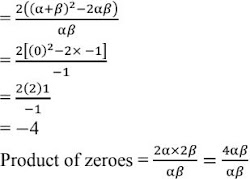Hence the quadratic equation is x2 - (sum of zeroes)x + product of zeroes
= k(x2 + 4x + 14)

16. If α and ß are the zeroes of the quadratic polynomial f(x) = x2 - 3x - 2, find a quadratic polynomial whose zeroes are 1/(2α + ß) + 1/(2ß+α).
Solution

f(x) = x2 - 3x - 2
Sum of zeroes [α +ß]  = 3
Product of zeroes [αß] = -2
Sum of zeroes = 1/[2α + ß] + 1/[2ß+α]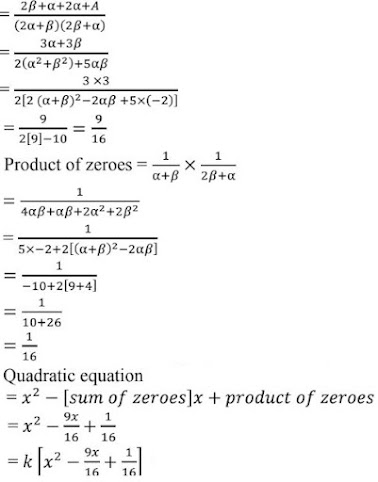17. If α and ß are the zeros of a quadratic polynomial such that α + ß  = 24 and α - ß = 8, find a quadratic polynomial having α and ß as its zeros.
Solution

α +ß = 24
αß = 8
.............
2α = 32
α = 16
ß = 8
αß = 16 × 8  = 128
⇒ x2 - (sum of zeroes) + product of zeroes
⇒ k[x2 - 24x + 128]

18. If α and ß are the zeroes of the quadratic polynomial f(x) = x2 - p(x+ 1) - c, show that (α +1)(ß + 1) = 1- c.
Solution

f(x) = x2 - p(x+1)c = x - px = -p -c
Sum of zeroes = α + ß = p
Product of zeroes = - p- c = αß
(α + 1+ß) = αß + α +ß +  1 = -p - c +p + 1
= 1 - c = R.H.S
∴ Hence, proved.

19. If α and ß are the zeros of the quadratic polynomial f(x) = x2 - 2x +3, find a polynomial whose roots are :
(i) α + 2, ß+2
(ii) (α - 1)/(α+1), (ß - 1)/(ß+1)
Solution

f(x) = x2 - 2x + 3
Sum of zeroes = 2 = (α + ß)
Product of zeroes  = 3 = (αß)

(i) sum of zeroes = (α + 2)+(ß+2) = α + ß+4 = 2+ 4 = 6
Product of zeroes = (α +2)(ß+2)
= αß+2α+2ß+4 = 3+2(2) + 4 = 11
Quadratic  equation = x2 - 6x + 11 = k[x2 - 6x + 11]

(ii) sum of zeroes = (α-1)/(α+1) + (ß-1)/(ß+1)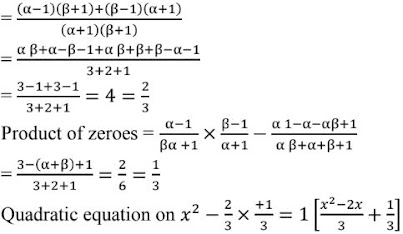20. If α and ß are the zeroes of the polynomial f(x) = x2 + px + q, form a polynomial whose zeroes are (α + ß)2 and (α - ß)2 .
Solution

f(x) = x2 + p +q
Sum of zeroes  = p = α + ß
Sum of the new polynomial = (α + ß)2 + (α - ß)2
= (-p)2  + α2  + ß2  - 2αß
= p2  + (α +  ß)2  - 2αß - 2αß
= p2 + p2 - 4q
= 2p2 - 4q
Product of zeroes = (α+ß)2 × (α - ß)2
= [-p]2 × (p2 - 4q) = (p2 - 4q)p2
Quadratic equation = x2 - [2p2 - 4q]+ p2 [-4q+ p]
f(x) = k{x2 - 2(p2 - 28)x + p2 (q2 - 4q)}